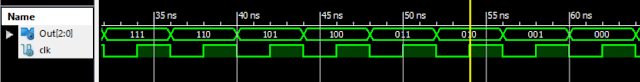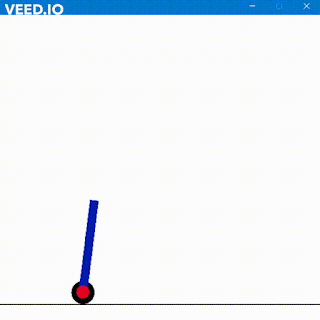### Verilog Code for MOD Counters

Counters are used to count and move the state of a circuit from one state to another. Whenever they are given a clock signal, either the system moves one state ahead or behind. It is not necessary to jump only one state. We can jump by a number of steps but for that, we would require circuitry. Counters are examples of sequential circuits. It requires a clock signal to function and states are changed at either positive edge of the negative edge of the clock. It is important to decide on the type of counter. We can design either an asynchronous counter or a synchronous counter. In an asynchronous counter, only the first flip-flop is dependent on the clock. The output of the first-floor shop act as a clock to the second flip-flop. Similarly,  the output of the second flip-flipflop will act as the clock for the third flip-flop and it goes on. On the other side in a synchronous counter, each flip-flop receives the clock signal at the same time. So we can conclude that asynchronous counters are slower than the synchronous counter. Asynchronous counters required less circuit free then synchronous counter. For asynchronous counters, all the data will change one after another and will follow On the other hand, with a synchronous counter, data will change at the very moment, the clock is triggered either at the positive edge or the negative edge.

Now as the titles suggest, we have to design Mod N counter Where N equals the number of the FFs. For example, if we have a MOD 2 counter hence it will go from 00 to 11. Here N denotes the number of flip-flops required to design the counter and will provide 2 to the power N States. Thus, we can easily make counters of 2, 4, 8, 16 states and so on.

To design MOD counters, I'll be using D flip-flop as they are most sophisticated and easy to use. To know about D flip-flops, you can follow this link.

MOD 4 Down Counter

For MOD 4 counter, we will require 2 FFs. To count the no of FFs required, use this formula

N <= 2n

N represents the number of states, n represents the number of  FFs required. MOD 4 counter will have 4 states from 00 to 11. It will have 2 flip-flops.

Verilog Code:

Output:

MOD 4 UP Counter

Verilog Code:
Output:

MOD 8 Down Counter

For MOD 8 counter, we will require 3 FFs. To count the no of FFs required, use this formula

N <= 2n

N represents the number of states, n represents the number of  FFs required. MOD 8 counter will have 8 states from 000 to 111 and then will again return to zero. It will have 3 flip-flops.

Verilog Code:

Output:MOD 8 Up Counter

Verilog Code:

Output:

Cheers.